Related Articles
ML | Principal Component Analysis(PCA)
• Last Updated : 07 Jul, 2018

Principal Component Analysis (PCA) is a statistical procedure that uses an orthogonal transformation which converts a set of correlated variables to a set of uncorrelated variables. PCA is a most widely used tool in exploratory data analysis and in machine learning for predictive models. Moreover, PCA is an unsupervised statistical technique used to examine the interrelations among a set of variables. It is also known as a general factor analysis where regression determines a line of best fit.

Module Needed:

 `import` `pandas as pd``import` `numpy as np``import` `matplotlib.pyplot as plt``import` `seaborn as sns``%``matplotlib inline`

Code #1:

 `# Here we are using inbuilt dataset of scikit learn``from` `sklearn.datasets ``import` `load_breast_cancer`` ` `# instantiating``cancer ``=` `load_breast_cancer()`` ` `# creating dataframe``df ``=` `pd.DataFrame(cancer[``'data'``], columns ``=` `cancer[``'feature_names'``])`` ` `# checking head of dataframe``df.head()`

Output: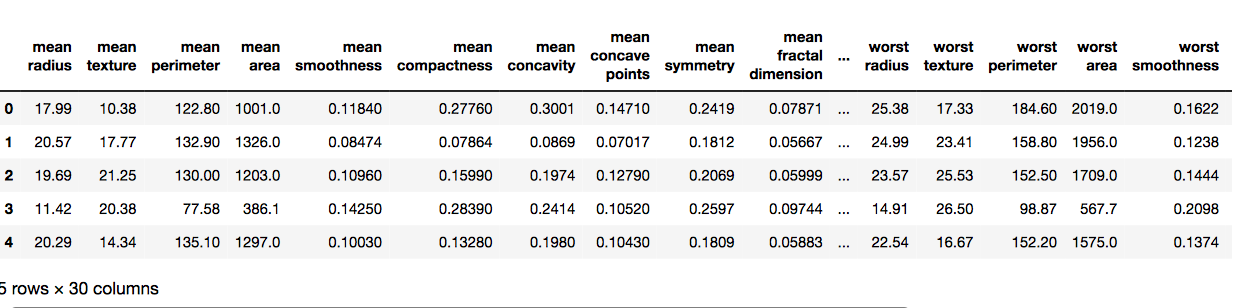Code #2:

 `# Importing standardscalar module ``from` `sklearn.preprocessing ``import` `StandardScaler`` ` `scalar ``=` `StandardScaler()`` ` `# fitting``scalar.fit(df)``scaled_data ``=` `scalar.transform(df)`` ` `# Importing PCA``from` `sklearn.decomposition ``import` `PCA`` ` `# Let's say, components = 2``pca ``=` `PCA(n_components ``=` `2``)``pca.fit(scaled_data)``x_pca ``=` `pca.transform(scaled_data)`` ` `x_pca.shape`

Output:
# Reduced to 569, 2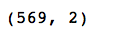`# giving a larger plot``plt.figure(figsize ``=``(``8``, ``6``))`` ` `plt.scatter(x_pca[:, ``0``], x_pca[:, ``1``], c ``=` `cancer[``'target'``], cmap ``=``'plasma'``)`` ` `# labeling x and y axes``plt.xlabel(``'First Principal Component'``)``plt.ylabel(``'Second Principal Component'``)`

Output: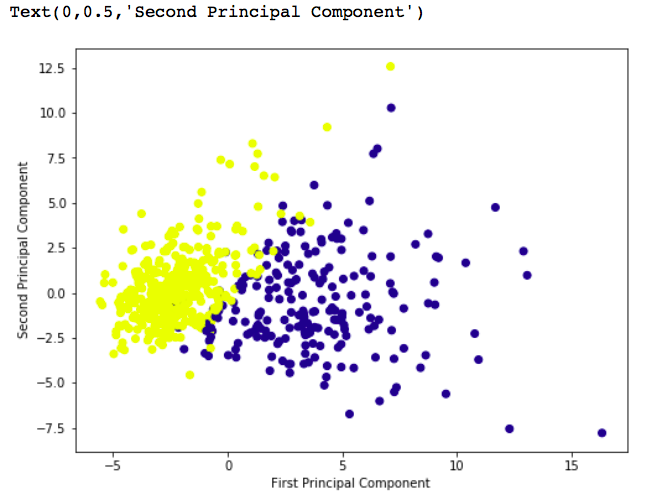`# components``pca.components_`

Output: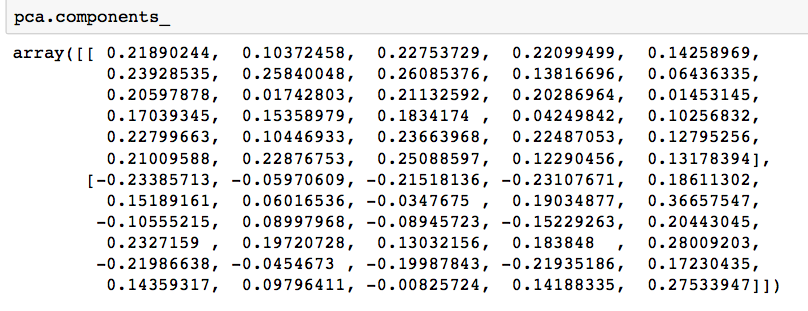`df_comp ``=` `pd.DataFrame(pca.components_, columns ``=` `cancer[``'feature_names'``])`` ` `plt.figure(figsize ``=``(``14``, ``6``))`` ` `# plotting heatmap``sns.heatmap(df_comp)`

Output: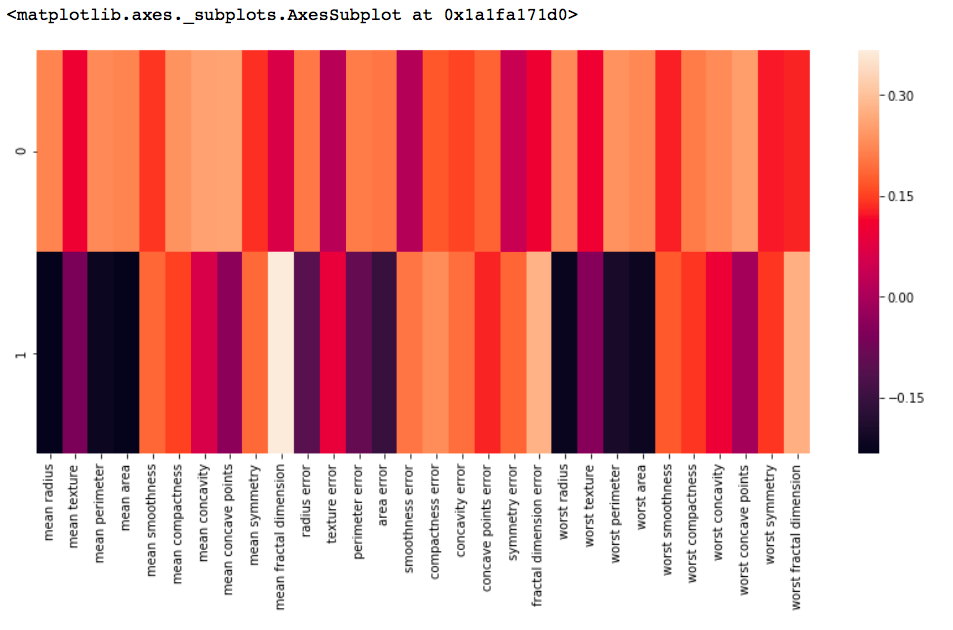My Personal Notes arrow_drop_up